# 智能驾驶功能软件平台设计规范第五部分：定位功能服务接口

0收藏

### 2 规范引用文件

 GB/T《汽车驾驶自动化分级》

 ISO/DIS 23150:2020(E) Road vehicles — Data communication between sensors

and data fusion unit for automated driving functions — Logical interface

 智能驾驶功能软件平台设计规范 第一部分：系统架构

 智能驾驶功能软件平台设计规范 第二部分：感知融合功能服务接口

 智能驾驶功能软件平台设计规范 第三部分：预测功能服务接口

 智能驾驶功能软件平台设计规范 第四部分：决策规划功能服务接口

### 3 缩略语

ODD Operational Design Domain 设计运行区域

APP Application 应用程序

### 4 定位功能概述●  绝对定位，是以世界坐标系为参考的定位输出，如以 WGS-84 坐标系为参考的自车位置（经度、纬度和高度）、姿态等信息。

●  相对定位，是以局部坐标系为参考的定位输出，如以局部地理系为参考的自车相对于局部参考系原点的位置、速度等信息。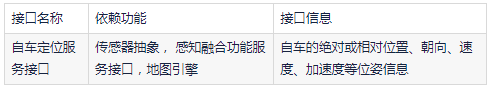### 5 定位功能服务接口定义

5.1 自车定位服务接口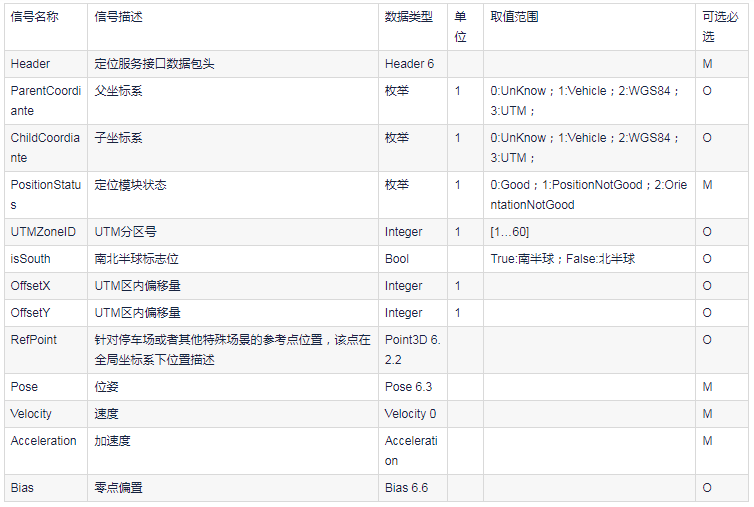### 6 定位功能服务接口数据结构

6.1 自车定位服务接口数据包头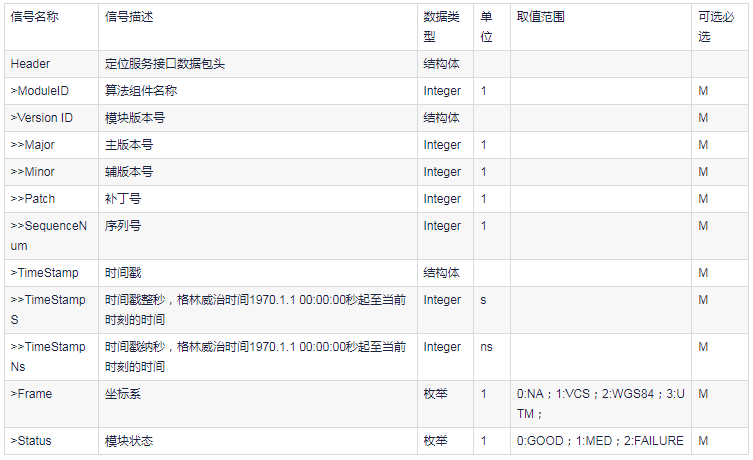6.2 描述

6.2.1 二维坐标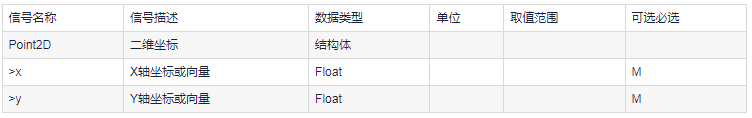6.2.2 三维坐标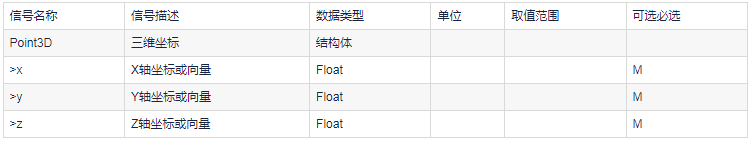6.2.3 二维曲线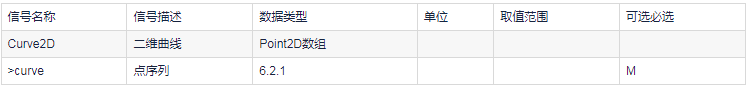6.2.4 三维曲线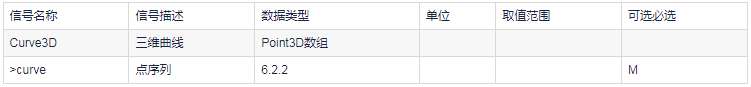6.2.5 二维多边形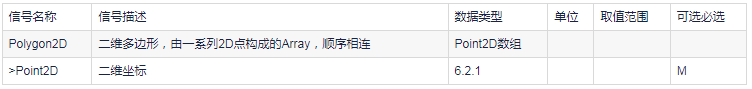6.2.6 三维多边形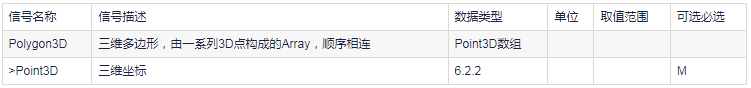6.2.7 四元数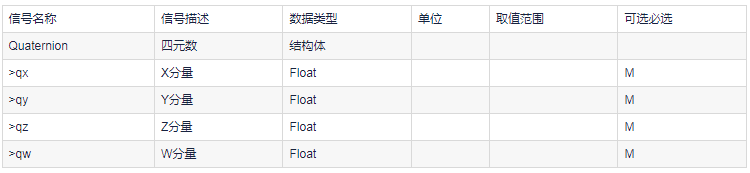6.3 位姿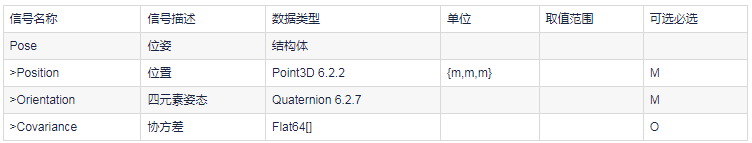6.4 速度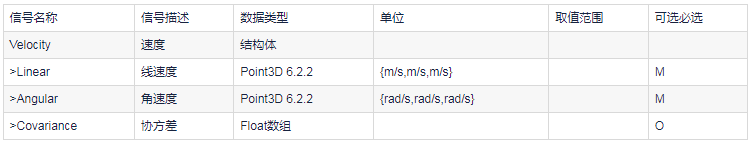6.5 加速度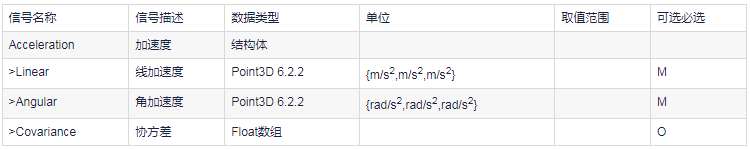6.6 零点偏置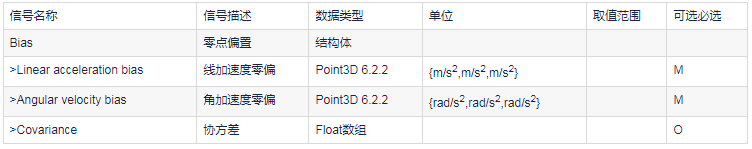### 附录A（资料性附录）定位功能服务接口Protobuf描述

A.1 数据结构定义

``````//common.proto
required uint32 ModuleID=1;
message VersionID{
required uint32 major=1;
required uint32 minor=2;
required uint32 patch=3;
}
required VersionID vid=2;
required uint32 sequenceNum=3;
message timeStamp{
required uint64 timeStampS=1;
required uint64 timeStampNs=2;
}
required timeStamp TimeStamp=4;
enum FRAMETYPE{
NA=0;
VCS=1;
WGS84=2;
UTM=3;
}
required FRAMETYPE Frame=5;
emum STATUS{
GOOD=0;
MED=1;
FAILURE=2;
}
required STATUS Status =6;
}
message Point2D{
required double x=1;
required double y=2;
}
message Point3D{
required double x=1;
required double y=2;
required double z=3;
}
message Quaternion {
Required double qx = 1 ;
Required double qy = 2 ;
Required double qz = 3 ;
Required double qw = 4 ;
}
Message Pose{
Required Point3D Position=1;
Required Quaternion quat=2;
Repeated double Covariance=3;
}
Message Velocity{
Required Point3D Linear=1;
Required Point3D Angular =2;
Repeated double Covariance=3;
}
Message Acceleration {
Required Point3D Linear=1;
Required Point3D Angular =2;
Repeated double Covariance=3;
}
Message Bias {
Required Point3D LinearAccelerationBias =1;
Required Point3D AngularVelocityBias =2;
Repeated double Covariance=3;
}``````

### A.2 自车定位服务接口描述

``````//LoacationService.proto
import common.proto
Message LoacationService{
optional FrameType ParentCoordinate=2;
optional FrameType ChildCoordinate=3;
Enum pStatus{
GOOD=0;
POSITION_NOT_GOOD=1;
ORIENTATIONNOTGOOD=2;
}
Required pStatus PositionStatus =4;
optional uint32 UTMZoneID=5;
optional bool IsSouth=6;
optional uint32 OffsetX=7;
optional uint32 OffsetY=8;
optional Point3D RefPoint=9;
Required Pose pose=10;
Required Velocity Vel=11;
Required Acceleration acc=12;
optional Bias imub=13;
}``````